PHY_10_37_V2_TG_Capacitors
Оценка 4.7

# PHY_10_37_V2_TG_Capacitors

Оценка 4.7
docx
07.05.2020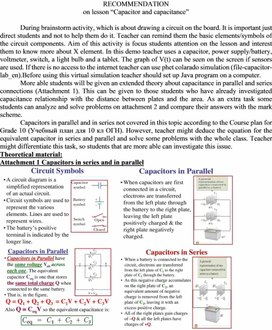PHY_10_37_V2_TG_Capacitors.docx

RECOMMENDATION

on lesson “Capacitor and capacitance”

During brainstorm activity, which is about drawing a circuit on the board. It is important just direct students and not to help them do it. Teacher can remind them the basic elements/symbols of the circuit components. Aim of this activity is focus students attention on the lesson and interest them to know more about X element. In this demo teacher uses a capacitor, power supply/battery, voltmeter, switch, a light bulb and a tablet. The graph of V(t) can be seen on the screen if sensors are used. If there is no access to the internet teacher can use phet colarado simulation (file-capacitor-lab_en).Before using this virtual simulation teacher should set up Java program on a computer.

More able students will be given an extended theory about capacitance in parallel and series connections (Attachment 1). This can be given to those students who have already investigated capacitance relationship with the distance between plates and the area. As an extra task some students can analyze and solve problems on attachment 2 and compare their answers with the mark scheme.

Capacitors in parallel and in series not covered in this topic according to the Course plan for Grade 10 (Учебный план для 10 кл ОГН). However, teacher might deduce the equation for the equivalent capacitor in series and parallel and solve some problems with the whole class. Teacher might differentiate this task, so students that are more able can investigate this issue.

Theoretical material:

Attachment 1 Capacitors in series and in parallel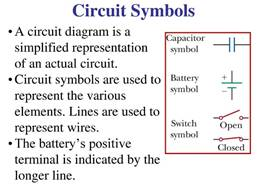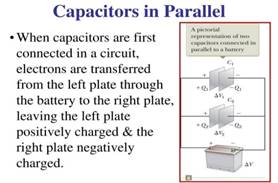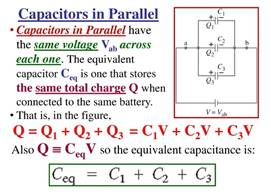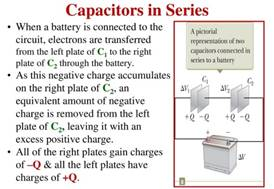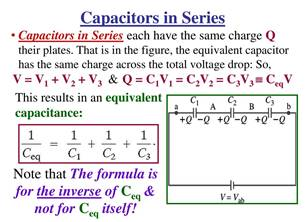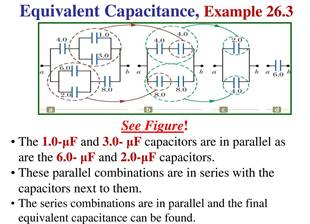Attachment 2 Problems with solution on topic Capacitor and capacitance

Problem solving. When solving problems, students follow the rule. Before proceeding to the next task, verify the solution with two neighbors.

1.                  Determine the charge accumulated by the capacitor on each plate of a 4.0 x 10-6 F capacitor when it is connected to a battery with a voltage of 12 V.

Answer: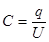; q = 48 mC

2.                  2. The distance between the plates of a flat capacitor 2.0 x10-3 m, determine the area of the plates, if the capacitor has a capacity of 1.0 nF.

Answer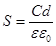; S = 0,225 m2

3.                  Calculate the voltage of the battery to which the capacitor is connected. The plate having an area of each plate is 2.0 cm2 and with a distance between the plates of 2 mm if the charge accumulated by the capacitor is 4.0 pC.

Answer:;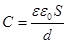;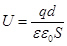;  U = 4.5 V

4.                  The area of each plate of a flat capacitor is 520 cm2. At what distance from each other should the plates be positioned so that the capacitance of the capacitor is 46 pF? Between the plates is leaf mica. The dielectric constant of mica is 7.

Answer:; d = 7 cm

1. Can we obtain two pieces of oppositely charged material when we place in the outer electric field

a) a piece of metal

b) a piece of polystyrene? Explain.

2. What will you observe when a piece of metal is placed into the electric field? Is there any attraction or repulsion?

3. What if you do the same with a piece of insulator? Is it the same or not? What is the difference?

4. Estimate the value of resultant electric field strength inside a conductor or insulator of relative permittivity 4 and finish the graphs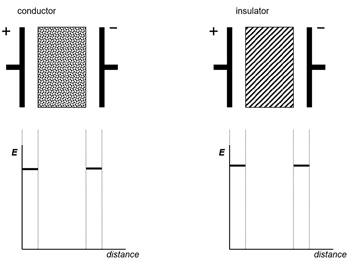5.    A 10 µF capacitor is connected to a 50 V supply. Calculate the charge and energy stored on the capacitor.

6.

a.    What is the capacitance of an empty parallel-plate capacitor with metal plates that each have an area of 1.00m21.00m2, separated by 1.00 mm?

b.    How much charge is stored in this capacitor if a voltage of 3.00×103V3.00×103V is applied to it?

Q=CV=(8.85×10−9F)(3.00×103V)=26.6μC.

7.    Suppose you wish to construct a parallel-plate capacitor with a capacitance of 1.0 F. What area must you use for each plate if the plates are separated by 1.0 mm?

1. A 30 μF capacitor is connected to a 9.0 V battery.

a Calculate the charge on the capacitor. 

b How many excess electrons are there on the negative plate of the capacitor?  (Elementary charge e = 1.6 × 10–19



2. The p.d. across a capacitor is 3.0 V and the charge on the capacitor is 150 nC. Determine the charge on the capacitor when the p.d. is:

a 6.0 V  b 9.0 V.



3. A 1000 μF capacitor is charged to a potential difference of 9.0 V. a Calculate the energy stored by the capacitor.  b Determine the energy stored by the capacitor when the p.d. across it is doubled.



4. For each circuit below, determine the total capacitance of the circuit.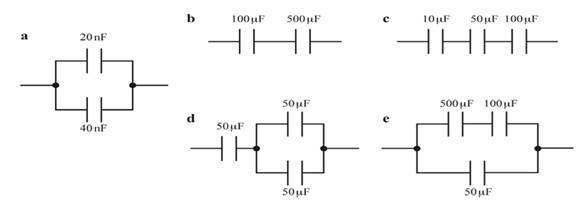5. The diagram shows an electrical circuit.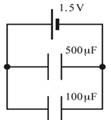6. A 10 000μF capacitor is charged to its maximum operating voltage of 32 V. The charged capacitor is discharged through a filament lamp. The flash of light from the lamp lasts for 300 ms.

a. Calculate the energy stored by the capacitor.



b. Determine the average power dissipated in the filament lamp.



7. The diagram shows a 1000 μF capacitor charged to a p.d. of 12 V. a Calculate the charge on the 1000 μF capacitor.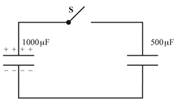b. The 1000μF capacitor is connected across an uncharged 500μF capacitor by closing the switch S. The charge initially stored by the 1000μF capacitor is now shared with  the 500μF capacitor. i Calculate the total capacitance of the capacitors in parallel.



ii Show that the p.d. across each capacitor is 8.0 V.



9 A 220 μF capacitor is charged to a potential difference of 8.0 V and then discharged through  a resistor of resistance 1.2 MΩ. a Determine the time constant τ of the circuit.



b Calculate: i the initial current in the circuit  ii the current in the circuit after a time equal to 2τ



iii the p.d. across the capacitor after a time of 50 s.



10. A 100 μF capacitor is discharged through a resistor of resistance 470 kΩ.

Determine the ‘half-life’ of this circuit. (The half-life of the circuit is the time taken for  the voltage across the capacitor to decrease to 50% of its initial value.)



11. The diagram below shows a charged capacitor of capacitance C. When the switch S is closed,  this capacitor is connected across the uncharged capacitor of capacitance 2C.

Calculate the percentage of energy lost as heat in the resistor and explain why the actual  resistance of the resistor is irrelevant.



Additional multiple choice questions: Capacitors (lower level)

47. Complete the following statement:  When a dielectric with constant k is inserted between the plates of a charged isolated capacitor

(a)        the capacitance is reduced by a factor k.

(b)       the charge on the plates is reduced by a factor of k.

(c)        the charge on the plates is increased by a factor of k.

(d)         the electric field between the plates is reduced by a factor of k.

(e)         the potential difference between the plates is increased by a factor of k.

48. A parallel plate capacitor has a potential difference between its plates of 1.2 V and a plate separation distance of 2.0 mm.  What is the magnitude of the electric field if a material that has a dielectric constant of 3.3 is inserted between the plates?

(a)         75 V/m                              (c)  250 V/m                            (e)  500 V/m

(b)         180 V/m                            (d) 400 V/m

49. A capacitor has a very large capacitance of 10 F.  The capacitor is charged by placing a potential difference of 2 V between its plates.  How much energy is stored in the capacitor?

(a)         2000 J                                (c)  100 J                                  (e)  20 J

(b)         500 J                                  (d) 40 J

50. The effective area of each plate of a parallel plate capacitor is 2.4 m2.  The capacitor is filled with neoprene rubber (k = 6.4).  When a 3.0-V potential difference exists across the plates of the capacitor, the capacitor stores 5.0 µC of charge.  Determine the plate separation of the capacitor.

(a)         7.2 ´ 10–5 m                      (c)  1.7 ´ 10–4 m                            (e)            8.2 ´ 10–5 m

(b)         3.0 ´ 10–4 m                      (d) 5.3 ´ 10–4 m

51. A uniform electric field of 8 V/m exists between the plates of a parallel plate capacitor.  How much work is required to move a +20 mC point charge from the negative plate to the positive plate if the plate separation is 0.050 m?

(a)        0.4 J                                   (c)  8 ´ 10–4 J                           (e)  8 ´ 10–6 J

(b)       1.6 J                                   (d) 8 ´ 10–5 J

52. A capacitor is initially charged to 2 V.  It is then connected to a 4 V battery.  What is the ratio of the final to the initial energy stored in the capacitor?

(a)        2                                        (c)  6                                        (e)  10

(b)       4                                        (d) 8

53. A parallel plate capacitor has plates of area 2.0 ´ 10–3 m2 and plate separation 1.0 ´ 10-4 m.

Determine the capacitance of this system if air fills the volume between the plates.

(a)        1.1 ´ 10–10 F                     (c)  3.2 ´ 10–10 F                           (e)            5.3 ´ 10–10 F

(b)       1.8 ´ 10–10 F                     (d) 4.4 ´ 10–10 F

54. A parallel plate capacitor has plates of area 2.0 ´ 10-3 m2 and plate separation 1.0 ´ 10-4 m.

Air fills the volume between the plates.  What potential difference is required to establish a

3.0 mC charge on the plates?

(a)         9.3 ´ 102 V                        (c)  1.7 ´ 104 V                              (e)            3.7 ´ 105 V

(b)         2.4 ´ 104 V                        (d) 6.9 ´ 103 V

55. A potential difference of 120 V is established between two parallel metal plates.  The magnitude of the charge on each plate is 0.020 C.  What is the capacitance of this capacitor?

(a)  170 µC                              (c)  7.2 µC                               (e)  2.4 C

(b)  24 µC                                (d) 0.12 C

Additional multiple choice questions: Capacitors (Average level)

The plates of a parallel plate capacitor each have an area of 0.40 m2 and are separated by a distance of 0.02 m.  They are charged until the potential difference between the plates is 3000 V.  The charged capacitor is then isolated.

56. Determine the magnitude of the electric field between the capacitor plates.

(a)         60 V/m                              (c)  1.0 ´ 105 V/m                    (e)  3.0 ´ 105 V/m

(b)         120 V/m                            (d) 1.5 ´ 105 V/m

57. Determine the value of the capacitance.

(a)         9.0 ´ 10-11 F                     (c)  3.6 ´ 10-10 F                           (e)                               6.4 ´ 10-10 F

(b)         1.8 ´  10-10 F                     (d) 4.8 ´ 10-10 F

58. Determine the magnitude of the charge on either capacitor plate.

(a)         1.8 ´ 10-7 C                      (c)  4.9 ´ 10-7 C                            (e)                               6.8 ´ 10-7 C

(b)         2.7 ´ 10-7 C                      (d) 5.4 ´ 10-7 C

59. How much work is required to move a –4.0 mC charge from the negative plate to the positive plate of this system?

(a)         –1.2 ´ 10-2 J                      (c)  –2.4 ´ 10-2 J                            (e)                               –5.4 ´ 10-2 J

(b)         +1.2 ´ 10-2 J                     (d) +2.4 ´ 10-2 J

60. Suppose that a dielectric sheet is inserted to completely fill the space between the plates and the  potential difference between the plates drops to 1000 V.  What is the capacitance of the system after the dielectric is inserted?

(a)         1.8 ´ 10-10  F                    (c)  5.4 ´ 10-10  F                          (e)                               6.8 ´ 10-10  F

(b)         2.7 ´ 10-10  F                    (d) 6.2 ´ 10-10  F

61. Suppose that a dielectric sheet is inserted to completely fill the space between the plates and the

potential difference between the plates drops to 1000 V.  Determine the dielectric constant.

(a)         0.333                                 (c)  3.0                                     (e)  2000

(b)         0.666                                 (d) 6.0

Useful resources:

https://isaacphysics.org/concepts/cp_capacitor

https://physics.info/capacitors/

https://opentextbc.ca/physicstestbook2/chapter/capacitors-in-series-and-parallel/

Скачано с www.znanio.ru

## RECOMMENDATION on lesson “Capacitor and capacitance”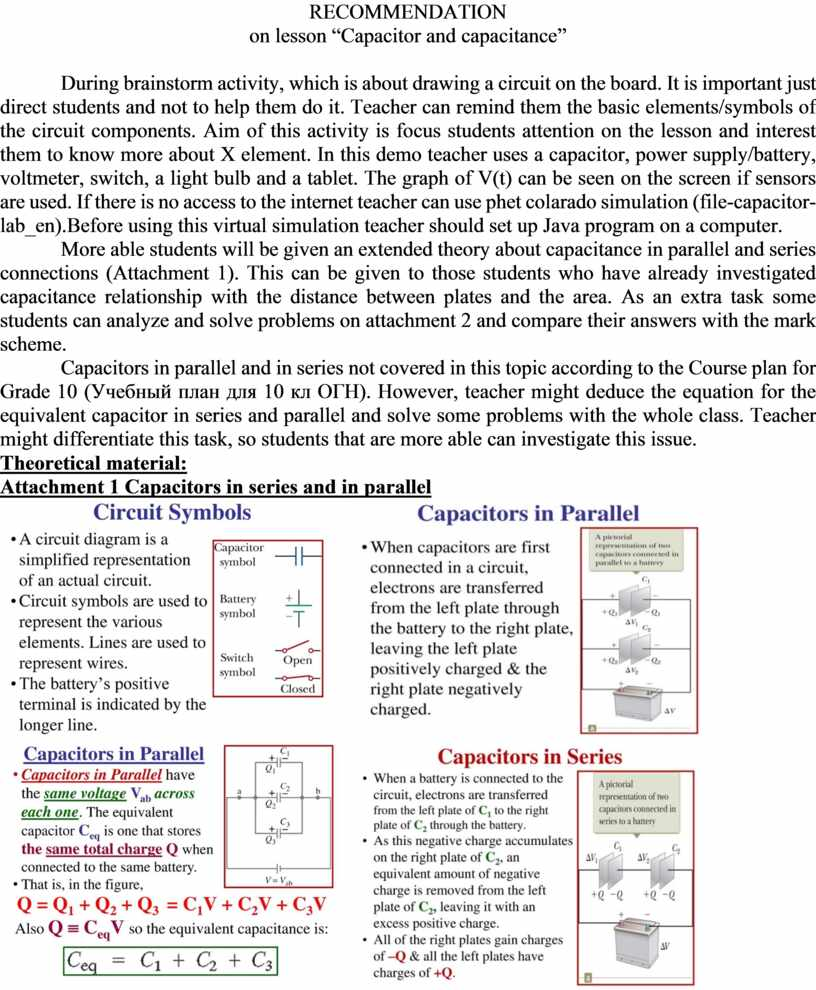## A 100 μF capacitor is discharged through a resistor of resistance 470 kΩ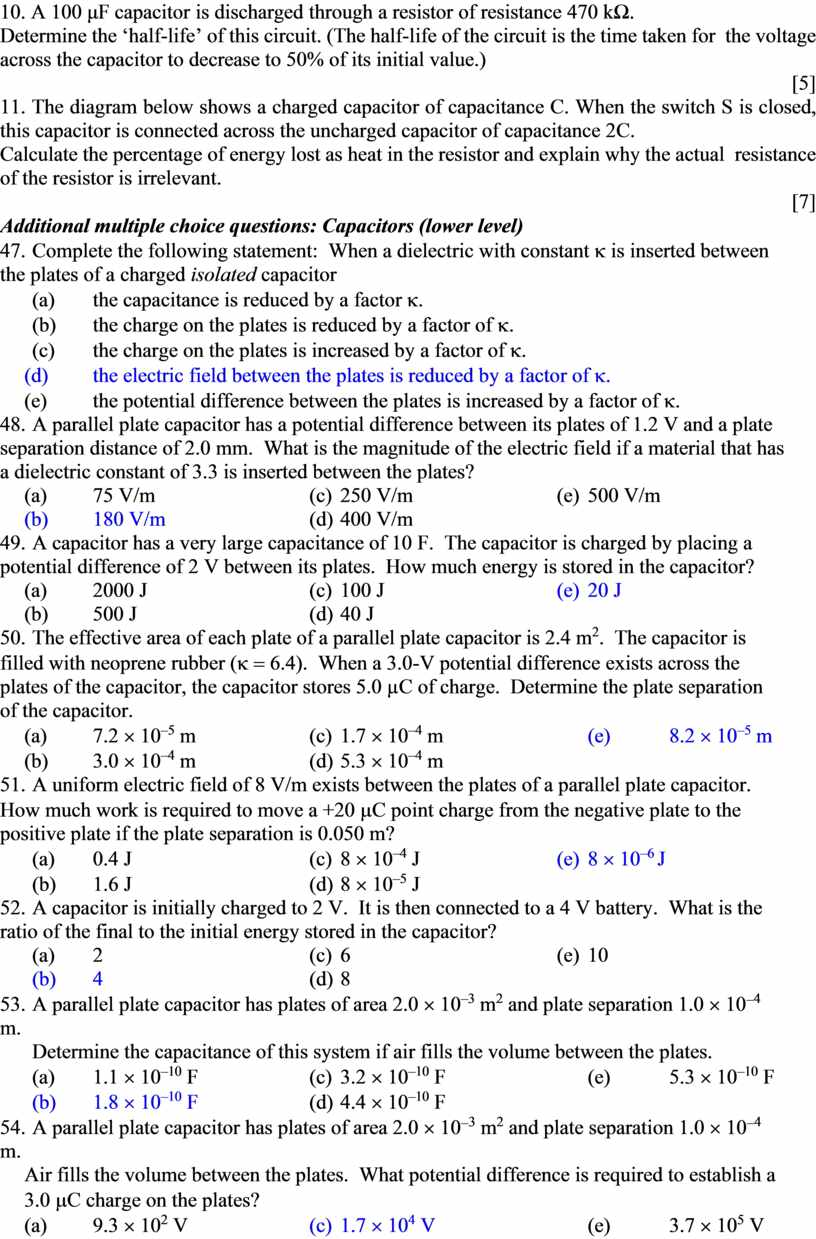Скачать файл